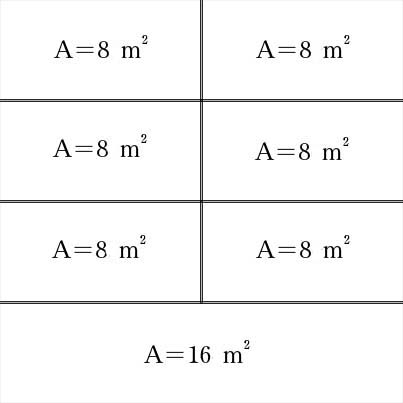SEARCH HOMEMath Central Quandaries & QueriesQuestion from anonomous, a student: I need to fit 7 different rectangles on grid paper of 1cm squared. Each squared centimeter equals 4 squared metres, and it needs to end up as area 64 square metres.If you want 1cm2 = 4m2 consider the following arrangement:The large rectangle is 8m x 2m and each of the small rectangles are 4m x 2m. This would require you to have widths that are 0.5cm for each rectangle, a length of 2 cm for the large rectangle and lengths of 1cm for each of the small rectangles.
JaniceMath Central is supported by the University of Regina and The Pacific Institute for the Mathematical Sciences.